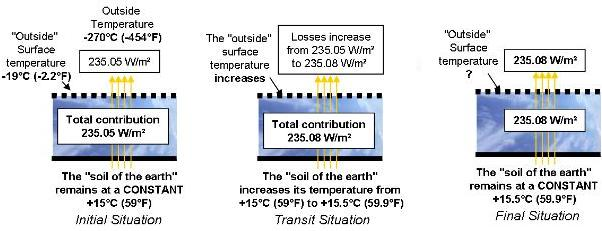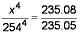Chargement...

# 6) Let’s assume global warming is not “observed” from space 6) Let’s assume global warming is not “observed” from space

6) Let’s assume global warming is not “observed” from space

Let’s assume that the current Global Warming is not “observed” from space and that the increase of 0.5 [°C] (0.9°F) at the surface was not observed as additional radiation transmitted towards space.Let’s compute under these conditions the “peripheral” temperature which our planet was nevertheless forced to reach in order to be able to evacuate the additional 0.03 [W/m²] brought about by human activity.

## Question

• Given that the flux exchanged by the planet with space prior to Global Warming amounts to 235.05 [W/m²] under an “exterior surface” temperature of -19 [°C] (-2.2°F) and space at -270 [°C] (-454°F).
• Given that the flux exchanged by radiation is of the following type:

Flux = k x (T14 - T24
With:
Flux: power exchanged by radiation in [W]
T1    : temperature of the warmer element in [K]
T2    : temperature of the colder element in [K]
k      : constant ratio in  [W/K4]

Determine the “exterior surface” temperature the earth must reach in order to be able to evacuate 235.08 [W/m²].

-18.992 [°C] (-2.1886°F), which corresponds to an increase in temperature of 0.008 [K].

Explanation:
Flux = k x (T14 – T24
With T1 and T2 expressed in Kelvin
T1 = -19 [°C] (-2.2°F) expressed in Kelvin,
T1 = -19 + 273 = 254 [K]
T2 = -270 [°C] (-454°F) expressed in Kelvin,
T2 = -270 + 273 = 3 [K]

Let X be the flux we are after.
We can write:
235.05 = k x (2544 - 34)
235.08 = k x (X4 - 34)

Ignoring the 34 term we can write:Hence X = 254.008 [K]
To evacuate 235.08 [W/m²] (instead of 235.05 [W/m²], the temperature of the exterior surface of the planet must change from -19 [°C] to -18.992 [°C] (-2.2°F to -2.1856°F) which corresponds to an increase in temperature of 0.008 [K].

Therefore, a minimal increase of 0.008 [K] of the “exterior surface” temperature of the planet is enough to evacuate the additional 1.5 x E13 [W] currently produced by humans. This minimal increase leads to a variation of flux emitted towards space from 235.05 [W/m²] to 235.08 [W/m²].

If, as we have previously seen, a power of 1.5 x E13 [W] can be enough to increase the surface temperature of the earth by 0.5 [K], it is possible only if this increase in temperature is not observed from space. Under these conditions, the increase in temperature difference between the surface and the “periphery” of the atmosphere could only be explained by an increase of its “thermal resistance” according to the definition given in the construction industry.

If the solar forcing from the past century, several tens of times greater than our current activity, led to only a few tenths of degrees temperature increase, it is because without variation of the thermal characteristics of the atmosphere, this increase of the surface temperature is completely sent back towards its periphery.

If Global Warming is not observed from space, the increase in temperature due to our activity should have been only 0.008 [K]…

Again, we need to observe that the consequence of our production (in terms of temperature increase) is 60 times greater than what it should have been.